# Shakespearian numbersDuring a recent party I got asked the question “Since$\pi$ has an infinite decimal expansion, does that mean the collected works of Shakespeare (suitably encoded) are in it somewhere?”

My first response was to point out that infinite decimal expressions are not enough: obviously$1/3=0.33333\ldots$ is a Shakespeare-free number (unless we have a bizarre encoding of the works in the form of all threes). What really matters is whether the number is suitably random. In mathematics this is known as the question about whether pi is a normal number.

If it is normal, then by the infinite monkey theorem then Shakespeare will almost surely be in the number. We actually do not know whether pi is normal, but it looks fairly likely. But that is not enough for a mathematician. A good overview of the problem can be found in a popular article by Bailey and Borwein. (Yep, one of the Borweins)

## Where are the Shakespearian numbers?

This led to a second issue: what is the distribution of the Shakespeare-containing numbers?

We can encode Shakespeare in many ways. As an ASCII text the works take up 5.3 MB. One can treat this as a sequence of 7-bit characters and the works as  37,100,000 bits, or 11,168,212 decimal digits. A simple code where each pair of digits encode a character would encode 10,600,000 digits. This allows just a 100 character alphabet rather than a 127 character alphabet, but is likely OK for Shakespeare: we can use the ASCII code minus 32, for example.

If we denote the encoded works of Shakespeare by$[Shakespeare]$, all numbers of the form$0.[Shakespeare]xxxxx\ldots$ are Shakespeare-containing.

They form a rather tiny interval: since the works start with ‘The’,$[Shakespeare]$ starts as “527269…” and the interval lies inside the interval$[0. 527269000\ldots , 0.52727]$, a mere millionth of$[0,1]$. The actual interval is even shorter.

But outside that interval there are numbers of the form$0.y[Shakespeare]xxxx\ldots$, where$y$ is a digit different from the starting digit of$[Shakespeare]$ and$x$ anything else. So there are 9 such second level intervals, each ten times thinner than the first level interval.

This pattern continues, with the intervals at each level ten times thinner but also 9 times as numerous. This is fairly similar to the Cantor set and gives rise to a fractal. But since the intervals are very tiny it is hard to see.

One way of visualizing this is to assume the weird encoding$[Shakespeare]=3$, so all numbers containing the digit 3 in the decimal expansion are Shakespearian and the rest are Shakespeare-free.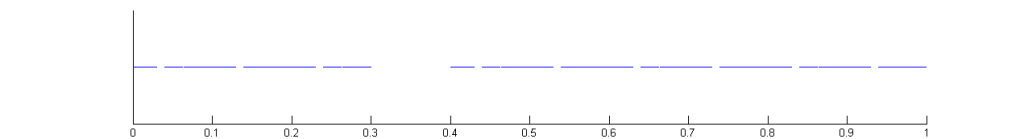Distribution of Shakespeare-free numbers in the unit interval, assuming Shakespeare’s collected works are encoded as the digit “3”.

The fractal dimension of this Shakespeare-free set is$\log(9)/\log(10)\approx 0.9542$. This is less than 1: most points are Shakespearian and in one of the intervals, but since they are thin compared to the line the Shakespeare-free set is nearly one dimensional. Like the Cantor set, each Shakespeare-free number is isolated from any other Shakespeare-free number: there is always some Shakespearian numbers between them.

In the case of the full 5.3MB [Shakespeare] the interval length is around$10^{-10,600,000}$. The fractal dimension of the Shakespeare-free set is$\log(10^{10,600,000} - 1)/\log(10^{10,600,600}) \approx 1-\epsilon$, for some tiny$\epsilon \approx 10^{-10,600,000}$.  It is very nearly an unbroken line… except for that nearly every point actually does contain Shakespeare.

We have been looking at the unit interval. We can of course look at the entire real line too, but the pattern is similar: just magnify the unit interval pattern by 10, 100, 1000, … times. Somewhere around  $10^{10,600,000}$ there are the numbers that have an integer part equal to$[Shakespeare]$. And above them are the intervals that start with his works followed by something else, a decimal point and then any decimals. And beyond them there are the$[Shakespeare][Shakespeare]xxx\ldots$ numbers…

## Shakespeare is common

One way of seeing that Shakespearian numbers are the generic case is to imagine choosing a number randomly. It has probability$S$ of being in the level 1 interval of Shakespearian numbers. If not, then it will be in one of the 9 intervals 1/10 long that don’t start with the correct first digit, where the probability of starting with Shakespeare in the second digit is$S$. If that was all there was, the total probability would be$S+(9/10)S+(9/10^2)S+\ldots = 10S<1$. But the 1/10 interval around the first Shakespearian interval also counts: a number that has the right first digit but wrong second digit can still be Shakespearian. So it will add probability.

Another way of thinking about it is just to look at the initial digits: the probability of starting with$[Shakespeare]$ is$S$, the probability of starting with$[Shakespeare]$ in position 2 is$(1-S)S$ (the first factor is the probability of not having Shakespeare first), and so on. So the total probability of finding Shakespeare is$S + (1-S)S + (1-S)^2S + (1-S)^3S + \ldots = S/(1-(1-S))=1$. So nearly all numbers are Shakespearian.

This might seem strange, since any number you are likely to mention is very likely Shakespeare-free. But this is just like the case of transcendental, normal or uncomputable numbers: they are actually the generic case in the reals, but most everyday numbers belong to the algebraic, non-normal and computable numbers.

It is also worth remembering that while all normal numbers are (almost surely) Shakespearian, there are non-normal Shakespearian numbers. For example, the fractional number$0.[Shakespeare]000\ldots$ is non-normal but Shakespearian. So is$0.[Shakespeare][Shakespeare][Shakespeare]\ldots$ We can throw in arbitrary finite sequences of digits between the Shakespeares, biasing numbers as close or far as we want from normality. There is a number$0.[Shakespeare]3141592\ldots$ that has the digits of$\pi$ plus Shakespeare. And there is a number that looks like$\pi$ until Graham’s number digits, then has a single Shakespeare and then continues. Shakespeare can hide anywhere.

In things of great receipt with case we prove,
Among a number one is reckoned none.
Then in the number let me pass untold,
Though in thy store’s account I one must be
-Sonnet 136

# My Newtonmass FractalI like the hyperbolic tangent function. It is useful for making sigmoid curves for neurons and fitting growth rates, it enables a cute minimal surface. So of course it should be iterated to make fractals! And there is no better way to celebrate Newtonmass than to make fractals!

As iteration formula I choose$z_{n+1} = f(z_n) = \tanh(cz_n)$ , where c is a multiplicative constant. Iterating some number like 1 and plotting its fate produces the following “Mandelbrot set” in the c-plane – the colours here do not denote the time until escape to infinity but rather where in the complex plane the point ended up, as a function of c. In a normal Mandelbrot set infinity is an attractive fixed point; here it is just one place in the (extended) complex plane like any other.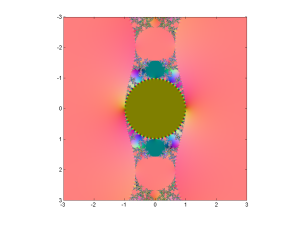“Mandelbrot set” for the hyperbolic tanh function tanh(cz).

The pinkish surroundings of the pattern represent points attracted to the positive solution of$z=\tanh(cz)$. There is of course a corresponding negative solution since tanh is antisymmetric: if z is an attractive fixed point or cycle, so is -z. So the dynamics is always bistable.

Incidentally, the color scheme is achieved by doing a stereographic projection of the complex plane onto a sphere, which is then fitted into the RBG cube. Infinity corresponds to (0.5,0.5,1) and zero to (0.5,0.5,0) – the brownish middle of the Mandelbrot set, where points are attracted towards zero for small c.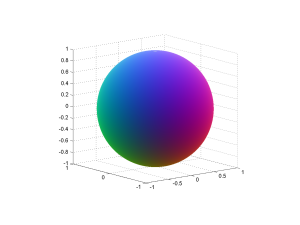Sphere used to stereographically map complex numbers to colors.

Another property of tanh is that the function has singularities wherever$z=\pm \pi n i / 2 c$ for integer$n>0$. Since Great Picard’s Theorem, that means that in the vicinity of those points it takes on nearly all other values in the complex plane. So whatever the pattern of the corresponding Julia set is, it will repeat itself near there (including images of the image, and so on).This means that despite most z points being attracted towards zero for c-values inside the unit circle, there will be a complex stitching of undefined points since they will be mapped to infinity, or are preimages of points that get mapped there.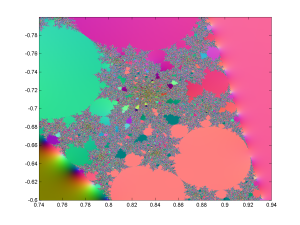Zoom into the tanh Mandelbrot set, showing chaotic regions with interspersed periodic regions.

Zooming into the messy regions shows that they are full of circle-cusp areas where there is a periodic attractor cycle. Between them are the regions where most of the z-plane where the Julia sets live is just pure chaos. Thanks to various classic theorems in the theory of complex iteration we know that if the Julia set has non-empty interior it is the entire complex plane.

Walking around the outside edge of the boring brown circle gives a fun sequence of patterns. At$c=1$ there are two real fixed points and a straight line border along the imaginary axis. This line of course contains the singularity points where things get sent to infinity, and near them the preimages of all the other singularities on the line: dramatic, but visually uninteresting.Tanh ‘Julia set’ for c=1.

As we move along the circle towards more imaginary c, there is a twisting of the border since each multiplication by c corresponds to a twist: it is now a fractal spiral covered by little spirals. As the twisting gets stronger, the spirals get bigger and wilder (especially when we are very close to the unit circle, where the dynamics has a lot of intermittency: the iterates almost but not quite gets stuck close to certain points, speed away, and then return to make rather elliptic spirals).Tanh ‘Julia set’ for c=1.1*exp(0.23*i).Tanh ‘Julia set’ for c=1.1*exp(0.5*i).Tanh ‘Julia set’ for c=1.1*exp(0.55*i).

When we advance towards a cuspy border in the c-plane we see the spirals unfold into long twisty tentacles just before touching, turning into borders between chains of periodic domains.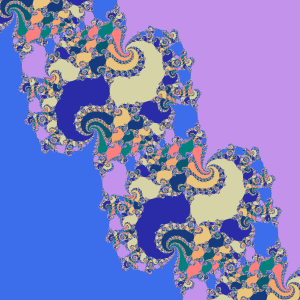Tanh ‘Julia set’ for c=1.1*exp(0.6*i).

But then the periodic domains start to snake out, filling the plane wildly.Tanh ‘Julia set’ for c=1.1*exp(0.6594*i).

until we get a plane-filling, ergodic Julia set with no discernible structure. For some c-values there are complex tesselations of basins of attraction, and quite often some places are close enough to weakly repelling fixed points to produce small circular false basins of attraction where divergence is slow.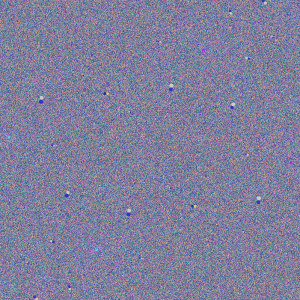Tanh ‘Julia set’ for c=1.1*exp(0.66*i).

One way of visualizing this is to make a bifurcation diagram like we do for real iteration. Following a curve$r e^{i\theta}$ we plot where iterates end up projected along some line (for example their real or imaginary part, or some combination). To make structure stand out a bit more I decided to color points after where in the whole plane they are, producing a colorful diagram for r=1.1:(I have some others on Flickr for the imaginary axis, r=1.25 and r=1.5).

Another, more fun way is to turn them into animated gifs. Since Flickr doesn’t handle them well, I have stored them locally instead:

• Growth of the Mandelbrot set – shows the behaviour of test iterates in the c-plane near the edge. Note the intermittent spirals.
• Unit circle – following the unit circle.
• Tanh 1.0 – the same as above, but inverted coordinates:$z=\infty$ is at the center, zero outside the borders.
• Tanh 1.1 – r=1.1.
• Tanh 1.5 – r=1.5.
• Tanh 2.5 – r=2.5.
• Tanh 5.0 – r=5.0. Rather sedate except for a brief window near$\theta=\pi/2$.

Note how spirals unfold until they touch each other, forming periodic domains or exploding across the entire plane, making a chaotic full-plane attractor… which often blinks into complex patterns of periodic domains only to return to chaos.# A sustainable orbital death rayI have for many years been a fan of the webcomic Schlock Mercenary. Hardish, humorous military sf with some nice, long-term plotting.

In the current plotline (some spoilers ahead) there is an enormous Chekov’s gun: Earth is surrounded by an equatorial ring of microsatellites that can reflect sunlight. It was intended for climate control, but as the main character immediately points out, it also makes an awesome weapon. You can guess what happens. That leds to an interesting question: just how effective would such a weapon actually be?

From any point on Earth’s surface only part of the ring is visible above the horizon. In fact, at sufficiently high latitudes it is entirely invisible – there you would be safe no matter what. Also, Earth likely casts a shadow across the ring that lowers the efficiency on the nightside.

I guessed, based on the appearance in some strips, that the radius is about two Earth radii (12,000 km), and the thickness about 2000 km. I did a Monte Carlo integration where I generated random ring microsatellites, checking whether they were visible above the horizon for different Earth locations (by looking at the dot product of the local normal and the satellite-location vector; for anything above the horizon this product must be possible) and were in sunlight (by checking that the distance to the Earth-Sun axis was more than 6000 km). The result is the following diagram of how much of the ring can be seen from any given location: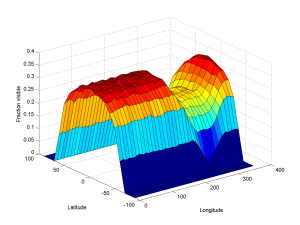Visibility fraction of an equatorial ring 12,000-14,000 km out from Earth for different latitudes and longitudes.

At most, 35% of the ring is visible. Even on the nightside where the shadow cuts through the ring about 25% is visible. In practice, there would be a notch cut along the equator where the ring cannot fire through itself; just how wide it would be depends on the microsatellite size and properties.

Overlaying the data on a world map gives the following footprint: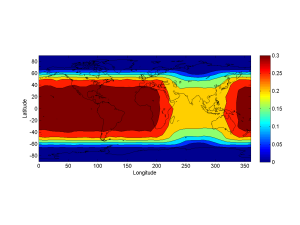Visibility fraction of 12,000-14,000 ring from different locations on Earth.

The ring is strongly visible up to 40 degrees of latitude, where it starts to disappear below the southern or northern horizon. Antarctica, northern Canada, Scandinavia and Siberia are totally safe.

This corresponds to the summer solstice, where the ring is maximally tilted relative to the Earth-Sun axis. This is when it has maximal power: at the equinoxes it is largely parallel to the sunlight and cannot reflect much at all.

The total amount of energy the ring receives is$E_0 = \pi (r_o^2-r_i^2)|\sin(\theta)|S$ where$r_o$ is the outer radius,$r_i$ the inner radius, $\theta$ the tilt (between 23 degrees for the summer/winter solstice and 0 for equinoxes) and$S$ is the solar constant, 1.361 kW/square meter. This ignores the Earth shadow. So putting in$\theta=20^{\circ}$ for a New Years Eve firing, I get$E_0 \approx 7.6\cdot 10^{16}$ Watt.

If we then multiply by 0.3 for visibility, we get 23 petawatts – is nothing to sneeze at! Of course, there will be losses, both in reflection (likely a few percent at most) and more importantly through light scattering (about 25%, assuming it behaves like normal sunlight). Now, a 17 PW beam is still pretty decent. And if you are on the nightside the shadowed ring surface can still give about 8 PW. That is about six times the energy flow in the Gulf Stream.How destructive would such a beam be? A megaton of TNT is 4.18 PJ. So in about a second the beam could produce a comparable amount of heat.  It would be far redder than a nuclear fireball (since it is essentially 6000K blackbody radiation) and the IR energy would presumably bounce around and be re-radiated, spreading far in the transparent IR bands. I suspect the fireball would quickly affect the absorption in a complicated manner and there would be defocusing effects due to thermal blooming: keeping it on target might be very hard, since energy would both scatter and reflect. Unlike a nuclear weapon there would not be much of a shockwave (I suspect there would still be one, but less of the energy would go into it).

The awesome thing about the ring is that it can just keep on firing. It is a sustainable weapon powered by renewable energy. The only drawback is that it would not have an ommminous hummmm….Addendum 14 December: I just realized an important limitation. Sunlight comes from an extended source, so if you reflect it using plane mirrors you will get a divergent beam – which means that the spot it hits on the ground will be broad. The sun has diameter 1,391,684 km and is 149,597,871 km away, so the light spot 8000 km below the reflector will be 74 km across. This is independent of the reflector size (down to the diffraction limit and up to a mirror that is as large as the sun in the sky).Intensity with three overlapping beams.

At first this sounds like it kills the ring beam. But one can achieve a better focus by clever alignment. Consider three circular footprints arranged like a standard Venn diagram. The center area gets three times the solar input as the large circles. By using more mirrors one can make a peak intensity that is much higher than the side intensity. The vicinity will still be lit up very brightly, but you can focus your devastation better than with individual mirrors – and you can afford to waste sunlight anyway. Still, it looks like this is more of a wide footprint weapon of devastation rather than a surgical knife.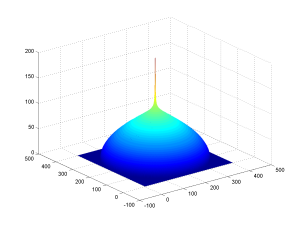Intensity with 200 beams overlapping slightly.

# Clashing discoursesOn Practical Ethics I blogged about Limiting the damage from cultures in collision, how clashing cultures of discourse can make a debate chaotic or even destructive. I took a bit of risk since the post dealt with things tangential to Gamergate, and I did indeed get some vigorous commenting – some of which was on target. A fair bit was a neat illustration of my thesis instead.

One interesting tip I got was from Adam Hyland about the paper 4chan and /b/: An Analysis of Anonymity and Ephemerality in a Large Online Community by Bernstein et al. They give some support for the ideas in the essay that started my post, how forums with high anonymity and ephemerality can produce very different discourse cultures. As some commenters in the twitter threat point out, however these forums also have methods of retaining memory – but it is a non-individual collective memory, rather a strict memory of who said what.

We can play around with how anonymous/pseudonymous/true nameish, ephemeral/permanent, quick/middle/long messages  are on a forum we build. It seems likely that somewhat predictable consequences  on the culture of discourse and how identity works would ensue: it would be a great project to test.